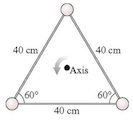# Problem: The three 210 g masses in the figure are connected by massless, rigid rods.a) What is the triangle’s moment of inertia about the axis through the center?b) What is the triangle’s kinetic energy if it rotates about the axis at 5.2 rev/s ?

###### Problem Details

The three 210 g masses in the figure are connected by massless, rigid rods.
a) What is the triangle’s moment of inertia about the axis through the center?
b) What is the triangle’s kinetic energy if it rotates about the axis at 5.2 rev/s ?Frequently Asked Questions

What scientific concept do you need to know in order to solve this problem?

Our tutors have indicated that to solve this problem you will need to apply the Intro to Rotational Kinetic Energy concept. You can view video lessons to learn Intro to Rotational Kinetic Energy. Or if you need more Intro to Rotational Kinetic Energy practice, you can also practice Intro to Rotational Kinetic Energy practice problems.

How long does this problem take to solve?

Our expert Physics tutor, Julia took 3 minutes and 39 seconds to solve this problem. You can follow their steps in the video explanation above.

What professor is this problem relevant for?

Based on our data, we think this problem is relevant for Professor Van Domelen's class at AMARILLO.